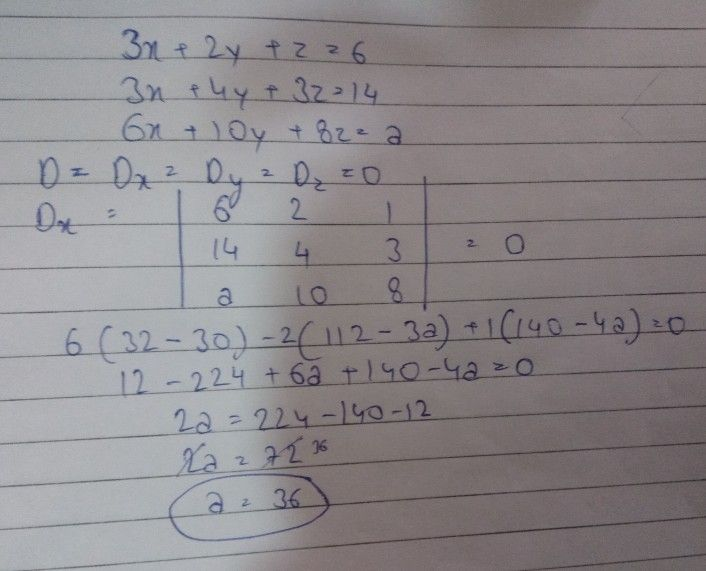Symbol
ProblemMATHEMATICS PHYSICS CHEMISTRY Question(7) The system of equations $3x+2y+z=6.3x+4y+3z=14$ $6x+10y+8z=a$ has infinite number of solutions, $11a=$ $A$ $8$ $B$ $12$ $24$ $D$ $36$
10th-13th grade
Calculus
Search count: 113
SolutionQanda teacher - Chris